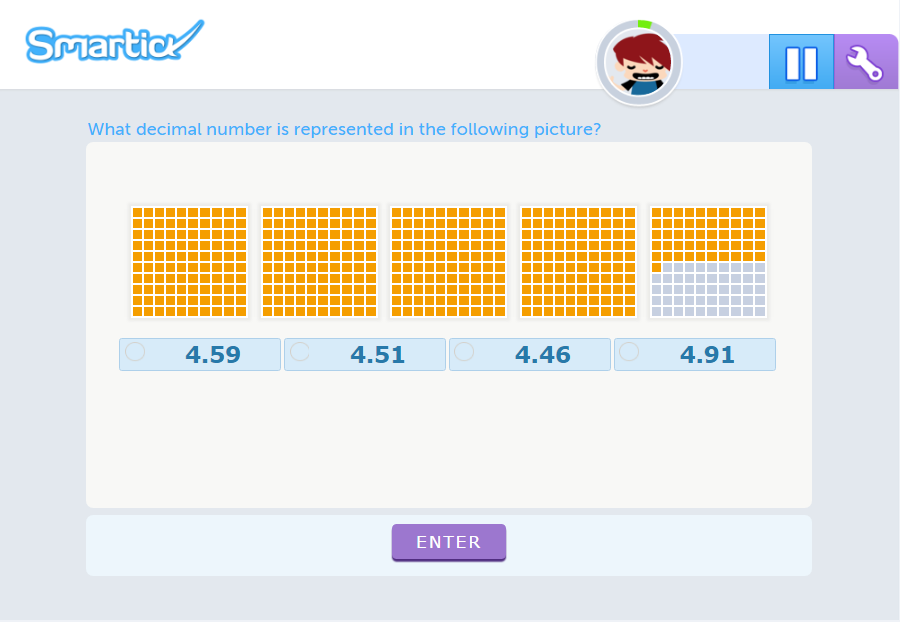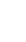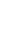Learning resources > DecimalsIntroduction
Through graphical representations, you'll understand the meaning of rational numbers, the positional structure of the decimal system and how to relate decimals to fractions.
Concept of decimals.
Basic level
PRACTICE EXERCISES »